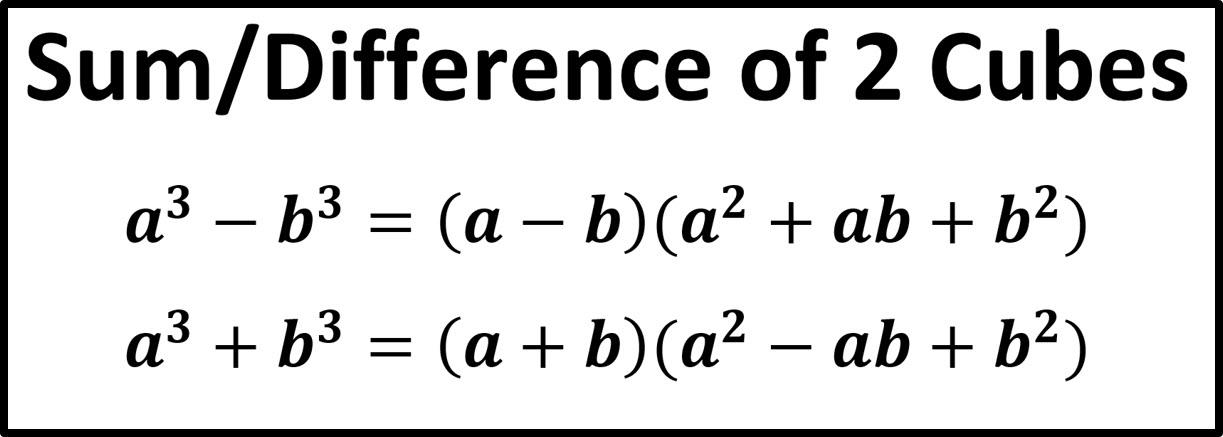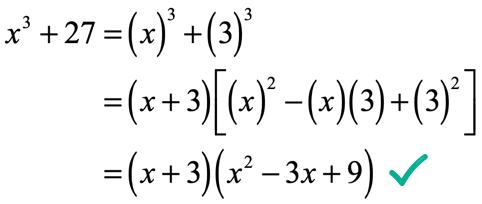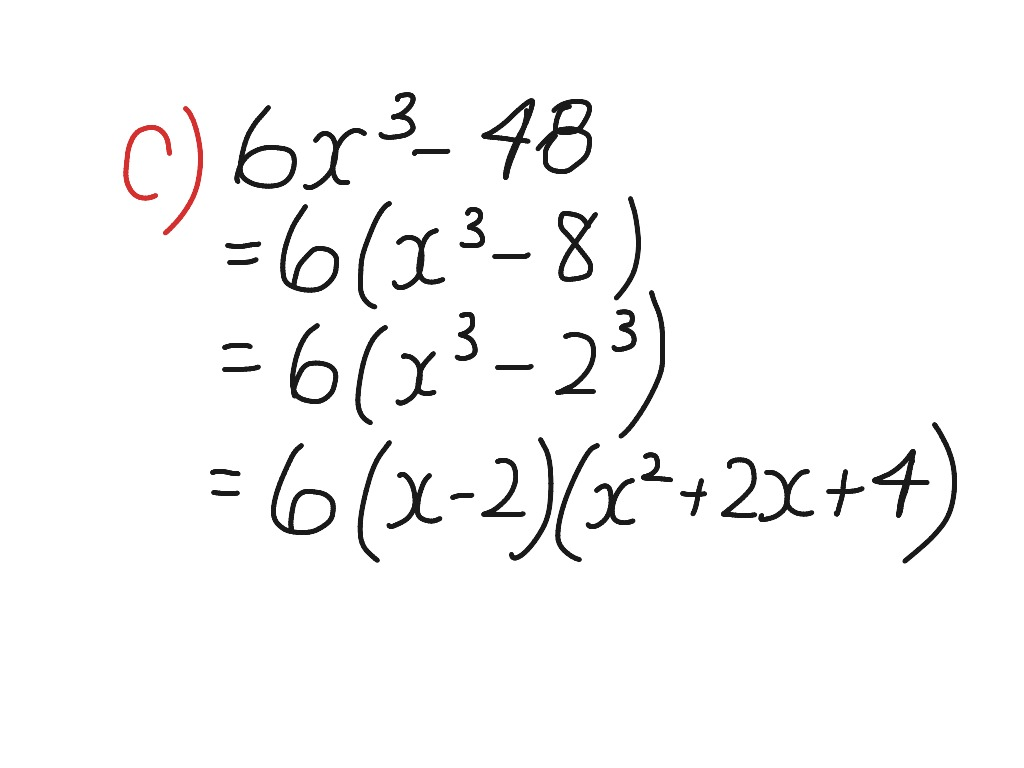# What are the Sums of Cubes ?

So, algebra is an integral part of our lives if you are into math or science. Like, it is the most basic thing. In middle school, we really start with algebra from which we delve into the deeper concepts. Therefore, no matter whatever concept we learn later on be it trigonometry, be it logarithms, be it exponents, or even calculus- algebra is something that you simply cannot forget about. Moreover, all of these find wide applications in math, physics, chemistry, economics- nearly everything. Hence, algebra is something in which your concepts must be clear such that you face absolutely no problem later on with the more difficult ones. However, if you are a newbie, remembering so many of the algebraic formulas like the sums of cubes, the sums of squares to begin with might not be very easy.Hence, let us first see what they mean, and then how we can represent or remember these formulae. So now, many of them might be very complicated apparently because these are things that you never use in arithmetic, that is all math you understand in primary school. However, nothing finds application as important and wide as algebra. So, this is all about given values, constants, and variables that you consider. Suppose, you need to share a huge amount amongst some people. Therefore, you can consider x amount that needs to be shared amongst y people. Now, the rules of algebra are not as simple as arithmetic. However, you can derive them all. So, coming to the sums of cubes- this is a formula that we will use to find the value of the addition of two entities in their cubes.

## Sums of Cubes formula

So, let us begin with the formula for the sums of cubes. However, when you are doing sums, there might be a number of ways you need to apply a formula. In every place, you cannot just apply the straightforward one. But, let us begin with the most basic one.

Therefore, let us consider “a” and “b” as the two entities whose cubes we are taking. Then, we will find the sum of cubes.So,

a^3 + b^3 = (a + b) (a^2 – ab + b^2)

Let us see if this works, that will help you to remember it. So, let us expand the right hand side of the formula.

Hence, (a + b) (a^2 – ab + b^2)

= a^3 – a^2 * b + b^2 * a + b * a^2 – b^2 * a + b^3

= a^3 + b^3, which is equal to the left hand side.

So, in sums, you might have the value of a + b and ab and you need to find the sum of cubes, that is a^3 + b^3.

However, from another point of view, depending on the kind of values you have at hand, the formula of the whole cube might also come in handy.

So, let us again consider that the two entities are “a” and “b”.

Hence, their whole cube would be (a + b)^3

So, (a + b)^3 = a^3 + 3a * b^2 + 3b * a^2 + b^3

Or, (a + b)^3 = a^3 + b^3 + 3ab (a + b)

Therefore, from here also you can find the sum of cubes. As per this one,

a^3 + b^3 = (a + b)^3 – 3ab (a + b)

## Sums of cubes calculator

The internet really makes everything way easier. So, there are sums of cubes calculators that you can use. Therefore, these might be helpful in two ways. First, they might help you solve sums when you cannot or when you are just starting out and need better understanding. Secondly, you might need it for verification of the answers that you have found manually. It often happens that the answers that we find manually are not correct. Moreover, sums of cubes might get very complex at times. The calculator shows all the steps precisely. So, if you have made a mistake in any of the steps, you can also quickly recognize it. Here, we will be considering the sums of cubes calculator from Symbolab.## Step 1 to use the sums of cubes calculator

So, to begin with, go to Symbolab and open the calculator. If you click on “Symbolab” above, you will directly land on their sums of cubes calculator. So, there are a number of functions at the top. Then there is a box in the shape of a search engine where you have to enter the data and at the bottom is the solution with the steps. Let us consider the expression to be x^3 + 27 that we need to factor.

## Step 2 to use the sums of cubes calculator

Hence, now we will have to feed in the data on which we are working. So, in the search-engine-like box, type x^3 + 27. Then click the red button right beside that reads “go” for the calculation to start.

## Step 3 to use the sums of cubes calculator

So, it takes a few seconds to calculate the sum of cubes and then you find the solution with all the steps. Therefore, at first, it shows the factored form from the question, that is (x + 3) (x^2 -3x +9). Then, it shows the steps.

Therefore, the first step is to transform the expression into cube form. That would be x^3 + 3^3.

So, now, the two terms are x and 3.

Therefore, x^3 + 3^3 = (x + 3) (x^2 – x * 3 + 3^2), as per the formula of sum of cubes.

Or, x^3 + 27 = x^3 + 3^3 = (x + 3) (x^2 -3x +9). So, there you get the final answer.

## Step 4 to use the sums of cubes calculator

Now, you can also check if your answer is correct in case you have another answer or you are not satisfied with the given answer. So, right below the solution segment, there is another box that looks like a search engine. Type your value there. Then click the red button that reads “verify” just beside and run.

## Sums of cubes examples

So, it is very difficult to understand algebra without the help of examples. We have already seen the formula for finding sums of cubes. Moreover, we have also seen how you can use a calculator to find it. Now, let us see a few examples to help you solve them manually.## 1.   Find the value of 50^3 + 60^3

So, here the two terms are 50 and 60. Now, you can individually cube 50 and 60 and then find their sum. However, that would be a tedious process if the values become larger and larger. Hence, we will solve it with the help of sums of cubes.

Therefore as per the formula,

50^3 + 60^3 = (50 + 60) (50^2 + 50 * 60 + 60^3)

Or, 50^3 + 60^3 = 110 * (2500 + 3000 + 3600)

So, 50^3 + 60^3 = 110 * 9100

Or, 50^3 + 60^3 = 1,001,000. So, this is the final answer.## 2.   Factor x^3 + 125

So, this example is a little different from the previous one. In the last one, you simply had to find the value of the sum of the cubes. In this case, you have to factor the expression as per the sum of the values or simplify it.

Therefore, as per the formula we have to reduce the expression in cube form at first.

So, x^3 + 125 = x^3 + 5^3

Therefore, the two terms here are x and 5.

So, x^3 + 5^3 = (x + 5) (x^2 + 5 * x + 5^2)

Or, x^3 + 5^3 = (x + 5) (x^2 + 5x + 25)

Hence, this is the final answer.

## Sums of cubes series

So, in this case, we are going to see the sums of cubes of natural numbers. Let us say, we consider n number of natural numbers. Therefore, Sn will be the sum of cubes till the nth term.

Hence,

Sn = 13 + 23 + 33 + 43 + … + n3 … (equation 1)

Now, the mother formula that we will use to derive this is n4 – (n – 1)4 = 4n3 – 6n2 + 4n – 1. Substituting the value of n as as 1, 2, 3, 4, … we get,

14 – 04 = 4 × 13 – 6 × 12 + 4 × 1 – 1

24 – 14 = 4 × 23 – 6 × 22 + 4 × 2 – 1

34 – 24 = 4 × 33 – 6 × 32 + 4 × 3 – 1

44 – 34 = 4 × 43 – 6 × 42 + 4 × 4 – 1

n4 – (n – 1)4 = 4 × n3 – 6 × n2 + 4 × n – 1

n4 – 04 = 4(13 + 23 + 33 + 43 + ……….. + n3) – 6 (12 + 22 + 32 + 42 + …….. + n2) + 4 (1 + 2 + 3 + 4 + …….. + n) – (1 + 1 + 1 + 1 + ……… n times).

Now, we use equation 1, the sum of squares and n natural numbers formula to get-

n4 = 4Sn – 6 × [n(n + 1)(2n + 1)/6] + 4 × [n(n+1)/2] – n

⇒ 4Sn = n4 + n(n + 1)(2n + 1) – 2n(n + 1) + n

⇒ 4Sn = n4 + n(2n2 + 3n + 1) – 2n2 – 2n + n

⇒ 4Sn = n4 + 2n3 + 3n2 + n – 2n2 – n

⇒ 4Sn = n4 + 2n3 + n2

⇒ 4Sn = n2 (n2 + 2n + 1)

⇒ 4Sn = n2 × (n + 1)2

⇒ Sn = [n2 × (n + 1)2]/4

So, if we substitute the value of Sn, we get, 13 + 23 + 33 + 43 + … + n3 = [n2 (n + 1)2]/4. Hence, this completes the proof of the sum of cubes of n natural numbers.## Sums of perfect cubes

Now, in all the above cases, wherever we have considered constants, they were all perfect cubes. So, if you cube root 27, you get a whole number- 3 or if you cube root 125, you get another whole number- 5. However, when you are considering x, it does not necessarily mean that the term would be a whole number. It can be a fraction as well. However, the sums of cubes formula apply perfectly well there also. So, the formula has little to do with the kind of values that you want to handle and the result depends on that. Read Also: Dimensional Analysis Calculator | Definition, Unit Converter & Examples

## Sums of cubes FAQs

### How do you identify a sum of cubes?

Ans. So, if you see a polynomial with cubes in the individual terms that are connected in addition, you will know it is a sum of cubes. To put it in simpler terms, let us consider the terms to be a and b. Cubing each we get a^3 and b^3. Now, if these two are added, it becomes a^3 + b^3. So, such an expression is a sum of cubes. Moreover, it is a polynomial because it has more than 1 algebraic term. However, it is not necessary that there will only be 2 terms. It can be more than that.

### Can a sum of squares be factored in?

Ans. Well yes, you can totally factor a sum of squares. Let us consider the individual terms to be again a and b. Therefore, the expression would be a^2 + b^2.

So, a^2 + b^2 = (a + b)^2 – 2abc. Let us see the derivation too.

Now, we all know-

(a + b)^2 = a^2 + b^2 + 2abc

So, from here if you shift sides, you can easily find the formula for the sum of squares.

### Can the sums or differences of two cubes be factored in?

Ans. So yes, you can factor the sum or difference of two cubes into simpler terms. Therefore, you simplify it into a binomial times trinomial. The symbols inside the binomial and trinomial will vary depending on whether it is sums of cubes that you are factoring or a difference of cubes. Check out the sums of cubes formula section above to get a better understanding of the factoring or simplification process.

### Which expression is a perfect cube?

Ans. So as we have already seen, a perfect cube is a value which if you cube root, you get a whole number. For example, if you cube root 64, you get 4. So, 4 x 4 x 4 makes 64 and 4 is a whole number. This makes 64 a perfect cube.